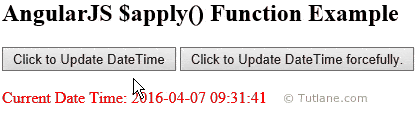# AngularJS \$apply() Function

Here we will learn \$apply() function in angularjs, use of \$apply function, difference between \$apply() and \$digest() functions in angularjs, using \$apply() function in angularjs applications with example.

## AngularJs \$apply() function

In angularjs \$apply() function is used to evaluate expressions outside of angularjs context (like browser DOM events, setTimeout, XHR or third party libraries). Generally in angularjs once \$apply() function execution finishes forcefully it will call \$digest() function to update all data bindings.

In angularjs the \$apply() function is a one of the central function of \$scope object.

## Difference between \$apply() vs. \$digest() Functions

Following are the differences between \$apply and \$digest() functions in angularjs.

1.  The \$apply() and \$digest() methods are used to update the model properties forcefully.

2.  The \$digest() method evaluate watchers for the current scope but \$apply() method is used to evaluate watchers for root scope that means it's for all scopes.

3.  The \$apply() method is used to update entire scope variables and bind model properties forcefully.

4.  The \$apply() method is slower than \$digest() method because \$digest() function trigger watchers on current \$scope but \$apply() function trigger watchers on entire scopes.

5.  If any error occurs in watch list while using \$digest() function it will not handle exceptions with \$expectionHandler service we need to write our own custom code to handle it but if we use \$apply() function internally it’s having try catch functionality to catch the errors while using watch list and send it to \$exceptionHandler service.

## Syntax of AngularJS \$apply() Function

Following is the syntax of using \$apply() function in angularjs applications.

\$scope.\$apply(function () {

});

We can use angularjs \$apply() function in different ways. Following are the different ways of using \$apply() function.

\$scope.\$apply(function () {

\$scope.val1 = 'tutlane.com';

});

// or

\$scope.\$apply('val1 = "tutlane.com"');

//or

\$scope.\$apply(function (scope) {

scope.val1 = 'tutlane.com';

});

We will see how to use \$apply() function in angularjs applications.

## Example of AngularJS \$apply() Function

Following is the example of using \$apply() function in angularjs applications.

<!DOCTYPE html>

<html>

<title>

AngularJs \$apply() Function with Example

</title>

<script type="text/javascript">

var app = angular.module('applyApp', []);

app.controller('applyCtrl', function (\$scope) {

\$scope.currentDateTime = new Date();

\$scope.updatedtime = function () {

\$scope.currentDateTime = new Date();

}

var event = document.getElementById("btnapply");

//The \$apply method is use to update date time on rootScope forcefully.

\$scope.\$apply(function () {

//get dateTime

\$scope.currentDateTime = new Date();

});

});

});

</script>

<body>

<div ng-app="applyApp" ng-controller="applyCtrl">

<h2>AngularJS \$apply() Function Example</h2>

<input type="button" value="Click to Update DateTime" ng-click="updatedtime()" />

<input type="button" value="Click to Update DateTime forcefully." id="btnapply" />

<br /><br />

<span style="color:Red">Current Date Time: {{currentDateTime | date:'yyyy-MM-dd HH:mm:ss'}}</span>

</div>

</body>

</html>

If you observe above code we are forcefully updating expression (\$scope.currentDateTime) value by calling \$apply() function on button click otherwise the changes whatever we made for expression (\$scope.currentDateTime) will not reflect in view of angularjs applications.

## Output of AngularJS \$apply() Function()

Following is the result of using \$apply() function in angularjs applications.This is how we can use \$apply() function in angularjs applications.